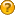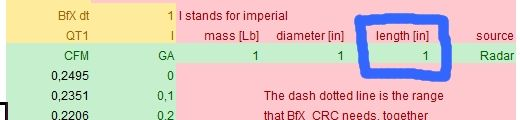###Author Topic: Ballistic coefficient and drag coefficient general questions  (Read 4776 times)

#### gvp

• Newbie
•• Posts: 17##### Ballistic coefficient and drag coefficient general questions
« on: June 17, 2013, 09:44:47 PM »
BC=Ballistic coefficient
Cd=Zero-yaw drag coefficient
M=projectile mass
D=Projectile diameter (caliber)
P=air pressure
T=air temperature
H=air humidity
shape=Geometric shape (form) of the projectile
u=projectile velocity
us=sound velocity at the projectile's atmosphere
u/us=projectile velocity/sound velocity ratio
SD=Projectile sectional density
i=form factor

Are the following points true or false ? (with the approximations used in BfX)

- us(P, dair)

- dair(P,T,H)     =>     us(P,T,H)

- BC(M, D, shape, u, P, T, H) or BC(M, D, shape, u/us)

- Cd(shape, u, P, T, H) or Cd(shape, u/us)

- SD=M/D2

- BC(M, D, shape, u/us)=SD/i(shape, u/us)

- i(shape, u/us)=Cdprojectile(shape, u/us)/Cdstandard projectile(u/us)

- Standard Gx projectiles always have diameter 1" and mass 1 lb

- A projectile with the exact same shape with the standard projectile (of the Gx drag function), but with different dimensions and weight, has a BC=SD at the same drag function, at all velocities

- Two projectiles with the same shape but with different dimensions and weights have the same Cd in the same velocity and at the same atmosphere.

- Lets say that we have a projectile with unknown BC ... the right way to build the BfX model for it is:
1. Compare the shape of the projectile with the Gx shapes and select the one more close to it.
2. Measure the BC near the muzzle (higher velocity with e.g. velocity differences at 2 points) according to Gx drag model selected in step 1.
3. Build the BfX model with the BC measured in step 2 and the drag function selected in step 1.

- Last question: why the BfX custom drag functions need as an input the length of the projectile ?« Last Edit: June 30, 2013, 10:16:55 PM by gvp »

• Full Member
•• Posts: 237##### Re: Ballistic coefficient and drag coefficient general questions
« Reply #1 on: July 08, 2013, 04:28:32 PM »

- us(P, dair)  BFX does not calculate the speed of sound, it always assumes that it has the value at Standard temp and pressure STP. This gives minor deviations with other computer codes. On the otherhand, the calculation of speed of sound introduces inaccuracies too, and I have never encountered a proper treatment of the subject. On the otherhand I did not look for it. I, personnally, can only guess why a drag function should be related to the mach number of a projectile.

- dair(P,T,H)     =>     us(P,T,H) - I  never looked into the matter. Wikipedia has an article about it.

- BC(M, D, shape, u, P, T, H) or BC(M, D, shape, u/us) the ballistic coefficient does not depend on air properties. It depends on all the physical properties of the projectile alone and is normalized on the properties of the projectile the bc's drag function belongs to. A first guess would be to normalize on the sectional density of the drag functions standard bullet, hence assuming the projectile has the same geometrical shape.

- Cd(shape, u, P, T, H) or Cd(shape, u/us) There is truth in both formula's. BFX uses standard drag functions at STP and gives with BfX_C a method to correct for air density effects.

The other questions I cannot answer now, I have to look in the books, it has been more than two years ago that I studied the subject

- Lets say that we have a projectile with unknown BC ... the right way to build the BfX model for it is:
the right way to determine the bc is to measure velocity as a function of distance and reproduce with bfx_vx the values. Use a fit procudure to find the optimum bc. you can download the excel files from my site.

Alternatively you can determine the Cd of a projectile also from these velocity measurements your selves. I have to look in the theory for the proper definitions.

- Last question: why the BfX custom drag functions need as an input the length of the projectile ?
I don't remember. I think I do not use the input, but require it for the sake of future developments. I have to look in the code.